# 前言

最近在学习人脸的目标检测任务时，用了Haar人脸检测算法，这个算法实现起来太简洁了，读入个.xml，调用函数就能用。但是深入了解我发现这个算法原理很复杂，也很优秀。究其根源，于是我找了好些篇相关论文，主要读了2001年Paul Viola和Michael Jones在CVPR上发表的一篇可以说是震惊了计算机视觉的文章，《Rapid Objection Dection using a Boosted Cascade of Simple Features》。这个算法最大的特点就是快！在当时，它能够做到实时演示人脸检测效果，这在当时的硬件情况下是非常震惊的，且还具有极高的准确率。同时在2011年，这篇论文在科罗多拉的会议上获得了“十年内影响最为深远的一篇文章”。在我们知道这篇文章有多么的NB之后，接下来我们来细细的品味这篇文章的技术细节。

• 第一个贡献是引入了“积分图”的图像表示方法，它能够加快检测时的计算速度；
• 第三个贡献是提出了注意力级联的算法，它能够让分类器更多聚焦于object-like区别，而不是与被检测目标无关的背景图像等区域，也是极大的加快了目标检测速度。

Haar分类器 = Haar-like特征 + 积分图法 + AdaBoost算法 + 级联

# 1. Haar-like特征提取

## 1.1 基本Haar特征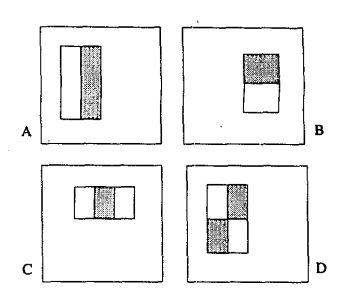$X = [\frac{W}{w}]\\ Y = [\frac{H}{h}]$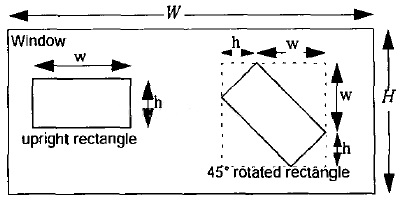$XY(W + 1-w\frac{X+1}{2})(H+1-h\frac{Y+1}{2})$

• 特征框竖直放大1倍，即无放大，竖直方向有(H-h+1)个特征
• 特征框竖直放大2倍，竖直方向有(H – 2h + 1)个特征
• 特征框竖直放大3倍，竖直方向有(H – 3h + 1)个特征
• 如此到竖直放大Y = floor(H/h)倍，竖直方向有1个特征，即(H-Y*h+1)

(H – h + 1) + (H – 2h + 1) + (H – 3h + 1) + …… + (H – Y*h + 1) = Y[H + 1 – h(1 + Y)/2]个特征。水平方向同理可得。

## 1.2 扩展Haar特征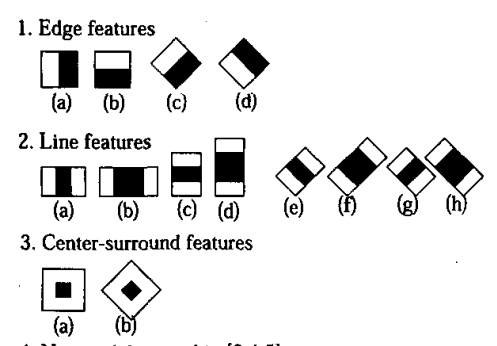## 1.3 Haar特征值的计算

$featureValue(x) = weight_{all} \times \sum_{pixel \in all}Pixel + weight_{black} \times \sum_{Pixel \in black} Pixel$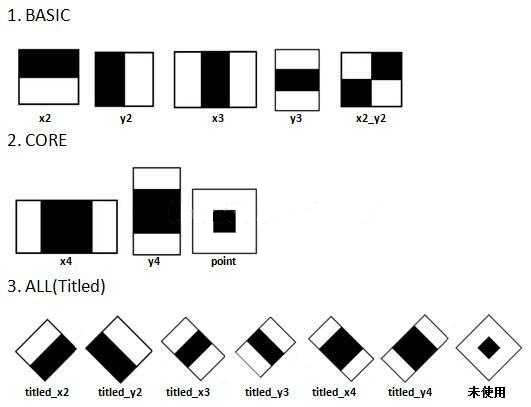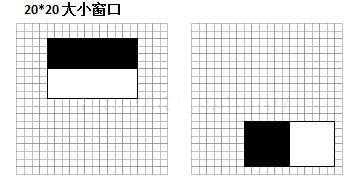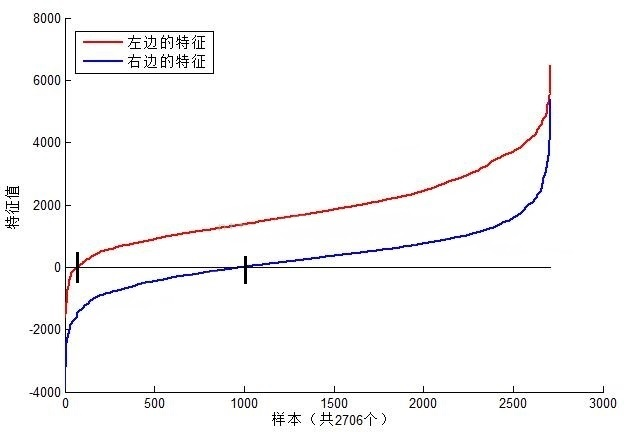• 在检测窗口通过平移 + 放大可以产生一系列的Haar特征（也就是说，黑白面积比例相同，但是大小不同的特征可以算作多个特征），这些特征由于位置和大小不同，分类效果也各异。
• 通过计算Haar特征的特征值，可以有效将图像矩阵映射为1维特征值，有效实现了降维。

## 1.4 Haar特征的保存

$(x \ y\ width \ height \ weight )$

## 1.5 Haar特征值标准化

• 计算检测窗口中间部分(w – 2) * (h – 2)的图像灰度值和灰度值平方和

$sum = \sum i(x,y), sqsum = \sum i^2(x,y)$

• 计算平均值：

$mean = \frac{sum}{w*h} \\ sqmean = \frac{sqsum}{w*h}$

• 计算标准化因子

• $varNormFactor = \sqrt{sqmean – mean^2}$

• 标准化特征值：

$normValue = \frac{featureValue}{varNormFactor}$

# 2. 积分图计算

$ii(i,j) = \sum_{k\leq i,l \leq j}f(k,l)$

1. 用s(i,j)表示行方向的累加和，初始化s(i,-1)=0;

2. 使用ii(i,j)表示一个积分图像，初始化ii(-1,i) = 0;

3. 逐行扫描图像，递归计算每个像素(i,j)行方向的累加和s(i,j)和积分图像ii(i,j)的值

$s(i,j) = s(i,j-1) + f(i,j) \\ ii(i,j) = ii(i-1.j) + s(i,j)$

4. 扫描图像一遍，当到达图像右下角像素时，积分图ii就构建好了。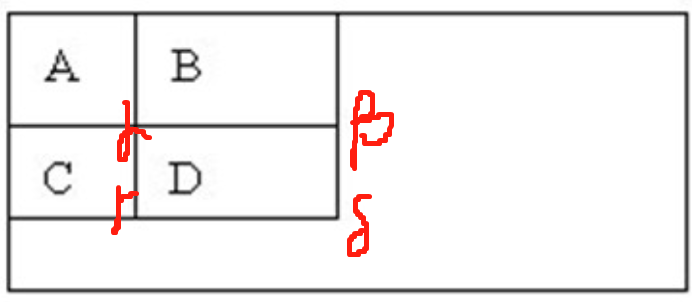$Dsum = ii(α) + ii(β) – (ii(γ)+ii(δ))$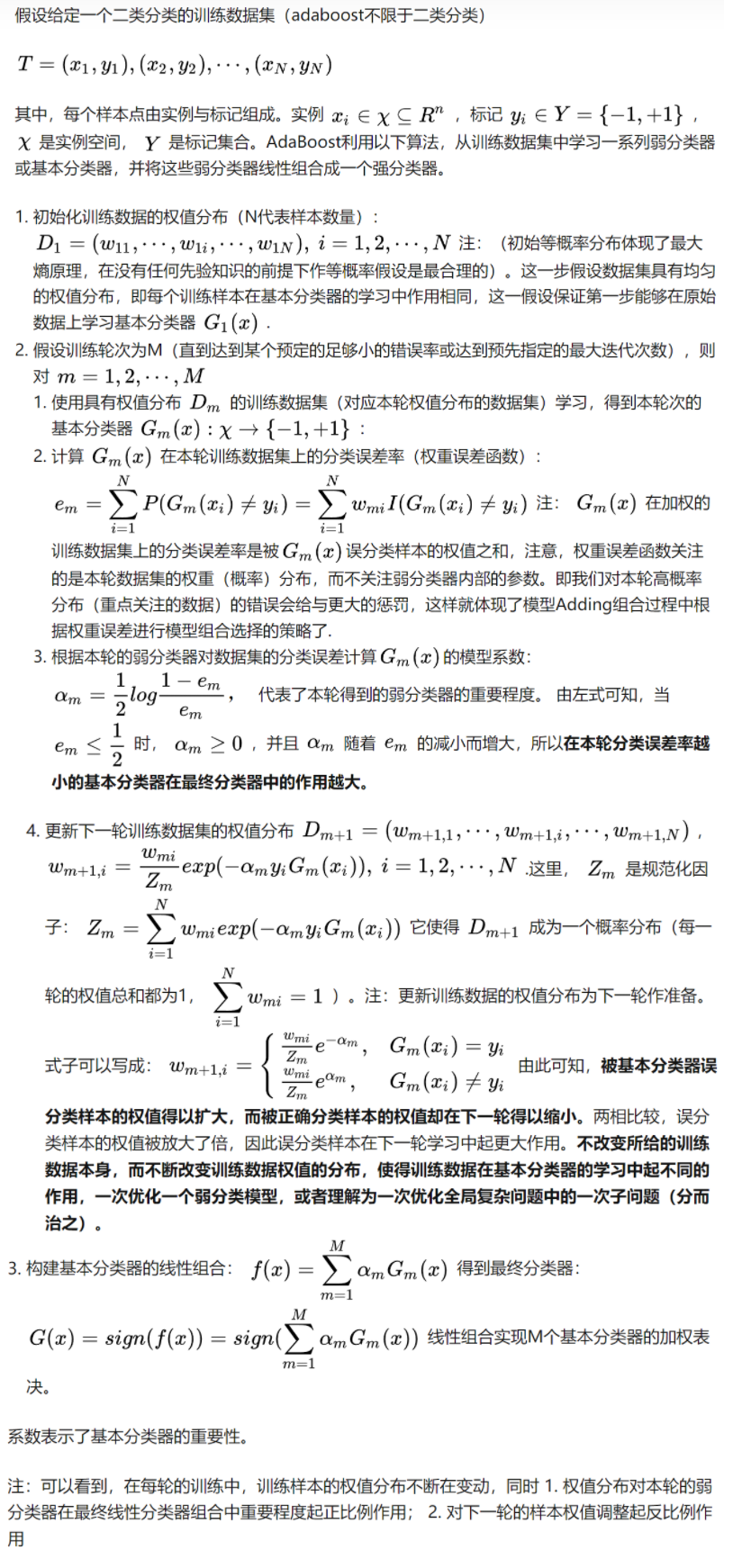## 4.1 弱分类器结构

Haar特征和弱分类器的关系很简单，一个完成的弱分类器包括：

• 若干个Haar特征 + 和Haar特征数量相等的弱分类器阈值
• 若干个LeftValue
• 若干个RightValue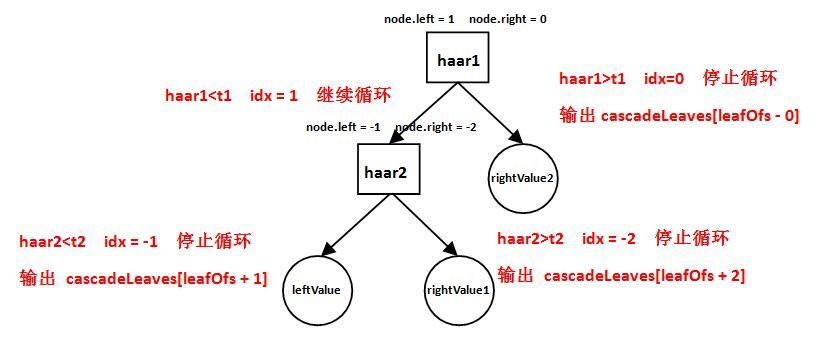• 计算第一个Haar特征的特征值haar1，与第一个弱分类器阈值t1对比，当haar1<t1时，进入步骤2；当haar1>t1时，该弱分类器输出rightValue2并结束。
• 计算第二个Haar特征值haar2，与第二个弱分类器阈值t2对比，当haar2<t2时输出leftValue；当haar2>t2时输出rightValue1。

## 4.2 强分类器结构

$sum += cascadeLeaves[LeafOfs – idx]$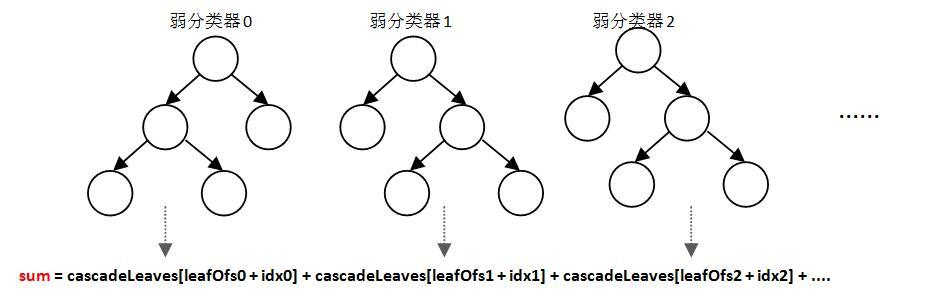1. 缩小图像就是把图像按照一定比例逐步缩小然后滑动窗口检测，如下图所示；

2. 放大检测窗口是把检测窗口长宽按照一定比例逐步放大，这时位于检测窗口内的Haar特征也会对应放大，然后检测。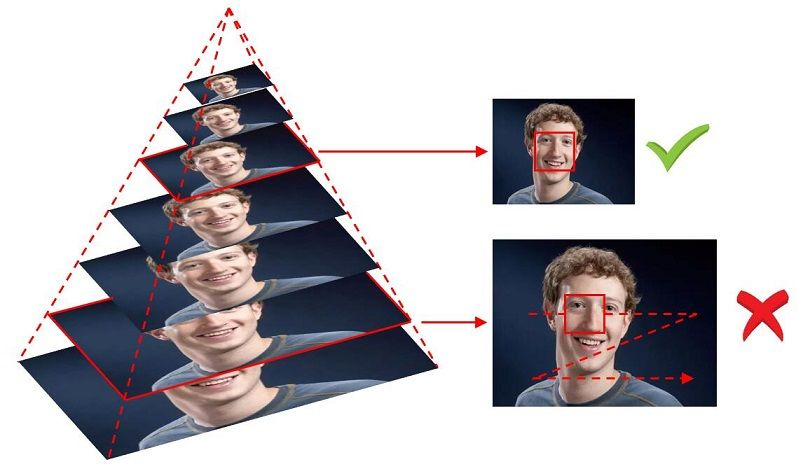## 4.4 级联分类器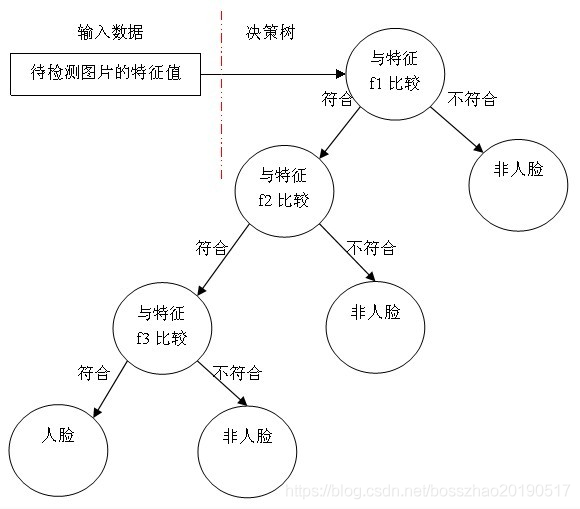## 4.5 级联分类器的训练

### 4.5.1 弱分类器的训练步骤

= h(x,f,ρ,Θ) =\left\{ \begin{aligned} 1，ρf(x)<ρΘ \\ 0，other \\ \end{aligned} \right.

1、对于每个特征 𝑓，计算所有训练样本的特征值，并将其排序：

2、扫描一遍排好序的特征值，对排好序的表中的每个元素，计算下面四个值：

• 计算全部正例的权重和𝑇+；

• 计算全部负例的权重和𝑇−；

• 计算该元素前之前的正例的权重和𝑆+；

• 计算该元素前之前的负例的权重和𝑆−；

• 选取当前元素的特征值$$F_{k,j}$$和它前面的一个特征值$$F_{k,j-1}$$之间的数作为阈值，所得到的弱分类器就在当前元素处把样本分开 —— 也就是说这个阈值对应的弱分类器将当前元素前的所有元素分为人脸（或非人脸），而把当前元素后（含）的所有元素分为非人脸（或人脸）。该阈值的分类误差为：

$e = min(S^+ + (T^- – S^-),S^- + (T^+ – S^+))$

### 4.5.2 强分类器的训练步骤

• 给定训练样本集$$x_i,y_i$$，i=1,2,4,5…N，共N个样本，$$y_i$$取值为0(负样本)或者1(正样本)；设正样本的数量为$$n_1$$，负样本的数量为$$n_2$$；T为训练的最大循环次数；

• 初始化样本权重为$$\frac{1}{n_1+n_2}$$，即为训练样本的初始概率分布

• for t = 1,…T:

• 权重归一化：

$w_{t,i} = \frac{w_{t,i}}{\sum_{j-1}^{n}w_{t,j}}$

• 对每一个特征j，训练一个分类器$$h_j$$；每个分类器只使用一种Haar特征进行训练，分类误差为:

$ε_j = \sum_i{w_j|h_j(x_i) – y_i|}$

• 从上一个步骤确定的分类器中，找出一个具有最小分类误差的弱分类器$$h_t$$

• 更新每个样本对应的权重

$w_{t+1,i} = w_t,i\beta_t^{1-e_i}$

如果样本$$x_i$$被正确分类，则$$e_i=0$$，否则$$e_i=1$$，而

$\beta_t = \frac{ε_t}{1-ε_t}$

• 最终形成的强分类器为：

h(x)=\left\{ \begin{aligned} 1,\sum_{t=1}^Tα_th_t(x) \geq \frac{1}{2}\sum_{t=1}^Tα_t \\ 0,otherwise \end{aligned} \right.

其中，$$α_t = log\frac{1}{\beta_t}$$

### 4.5.3 强分类器级联及训练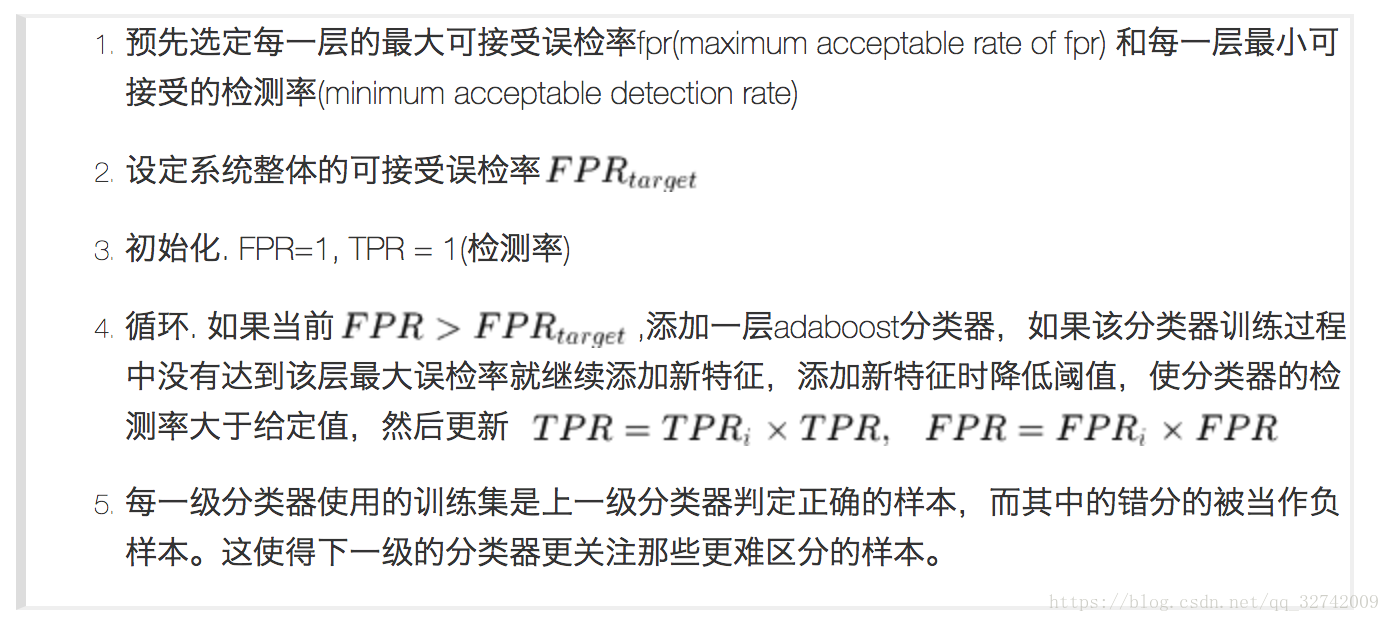$TruePositiveRate = \frac{TP}{TP+FN}，代表将真实正样本划分为正样本的概率\\ FalsePositiveRate = \frac{FP}{FP+TN}，代表将真实负样本划分为正样本的概率$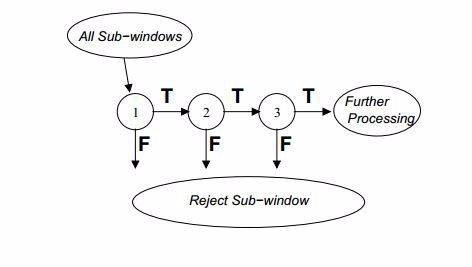1. 寻找TP和FP作为训练样本
2. 计算每个Haar特征在当前权重下的Best split threshold+leftvalue+rightvalue，组成了一个个弱分类器
3. 通过WSE寻找最优的弱分类器
4. 更新权重
5. 按照minHitRate估计stageThreshold
6. 重复上述1-5步骤，直到falseAlarmRate到达要求，或弱分类器数量足够。停止循环，输出stage。
7. 进入下一个stage训练

# 5. 总结

~：如果您发现文章中有出错的地方，欢迎您指正。同时也欢迎您在留言区讨论问题，共同学习。联系可E-mail:graywei2001@163.com.

# 6. 参考文献

 Haar-like特征提取原理

 OpenCV AdaBoost + Haar目标检测技术内幕（上）

 OpenCV AdaBoost + Haar目标检测技术内幕（下）

 Python+OpenCV图像处理（九）——Haar特征描述算子

 Face Detection with Haar Cascade

 Face Detection with Haar Cascade — Part II

 Rapid Object Detection using a Boosted Cascade of Simple Features中英版